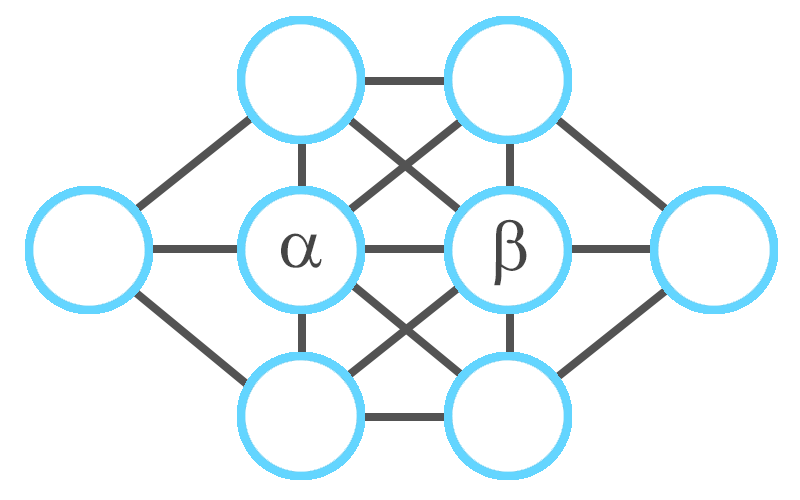# Christmas Streak 04/88: Fill In The Diagram

Logic Level 1

I want to fill in the eight circles below using each of the numbers 1, 2, 3, 4, 5, 6, 7, 8 exactly once. Additionally, consecutive numbers--e.g. $(1, 2)$ or $(5,6)$-- cannot be placed in circles which are connected by a line segment.

Is this possible, and if so, what is the sum of the two numbers in the middle?×